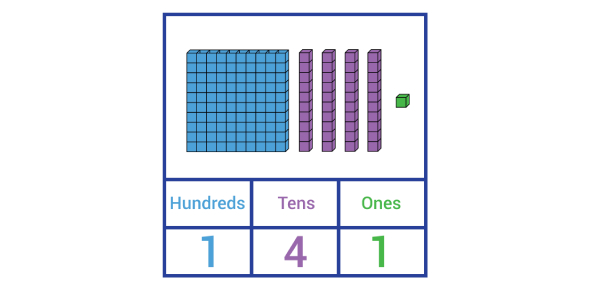# Rounding To Tens And Hundreds Quiz

Approved & Edited by ProProfs Editorial Team
At ProProfs Quizzes, our dedicated in-house team of experts takes pride in their work. With a sharp eye for detail, they meticulously review each quiz. This ensures that every quiz, taken by over 100 million users, meets our standards of accuracy, clarity, and engagement.
| Written by Alfredhook3
A
Alfredhook3
Community Contributor
Quizzes Created: 3190 | Total Attempts: 2,044,086
Questions: 10 | Attempts: 1,443SettingsHow strong your basic mathematics is? Are you good at rounding off numbers to tens and hundreds? If you're confident enough, then play this quiz and test your knowledge for the same. You can easily pass this quiz if you know the rounding tens or hundreds rules. For the nearest ten, the digit in the ones place is considered, whereas the tens place digit is considered to round a number to the nearest hundred. Shall we begin the quiz now? Best of luck!

• 1.

### Round 253 to the nearest tens.

• A.

240

• B.

300

• C.

250

• D.

200

C. 250
Explanation
To round 253 to the nearest tens, we look at the ones digit, which is 3. Since 3 is less than 5, we round down. Therefore, the tens digit remains the same, and the ones digit becomes 0. Hence, the number 253 rounds to 250.

Rate this question:

• 2.

### Round 817 to the nearest hundreds.

• A.

810

• B.

800

• C.

820

• D.

900

B. 800
Explanation
To round 817 to the nearest hundreds, we look at the tens digit, which is 1. Since it is less than 5, we round down the hundreds digit, which is 8, to the nearest hundreds. Therefore, the correct answer is 800.

Rate this question:

• 3.

### What digit do you need to look at to round 462 to the nearest tens?

• A.

4

• B.

6

• C.

2

• D.

None of these

C. 2
Explanation
To round 462 to the nearest tens, we need to look at the digit in the ones place, which is 2. Since 2 is less than 5, we do not need to round up. Therefore, the digit we need to look at to round 462 to the nearest tens is 2.

Rate this question:

• 4.

### What is 296 rounded to the nearest hundreds?

• A.

300

• B.

200

• C.

290

• D.

260

A. 300
Explanation
296 rounded to the nearest hundreds is 300 because when rounding to the nearest hundreds, we look at the tens digit. If it is 5 or greater, we round up, and if it is less than 5, we round down. In this case, the tens digit is 9, which is greater than 5, so we round up to the next hundred, which is 300.

Rate this question:

• 5.

### What is 781 rounded to the nearest tens?

• A.

700

• B.

710

• C.

800

• D.

780

D. 780
Explanation
The number 781, when rounded to the nearest tens, would be 780. This is because the digit in the ones place, which is 1, is less than 5. Therefore, we round down to the nearest tens, which is 780.

Rate this question:

• 6.

### Which of the following numbers is a weak number?

• A.

9

• B.

5

• C.

4

• D.

8

C. 4
Explanation
A weak number is a number that can be expressed as the sum of its own digits raised to the power of the number of digits. In this case, 4 is a weak number because it has only one digit, and 4^1 equals 4.

Rate this question:

• 7.

### Which of the following numbers is a strong numbers?

• A.

0

• B.

2

• C.

5

• D.

3

C. 5
Explanation
A strong number is a number whose sum of the factorial of its digits is equal to the number itself. In this case, the number 5 is a strong number because the factorial of its digit (5!) is equal to the number itself (5).

Rate this question:

• 8.

### What is 77 rounded to the nearest 10?

• A.

70

• B.

60

• C.

50

• D.

80

D. 80
Explanation
The number 77 is closer to 80 than to any other multiple of 10. When rounding to the nearest 10, if the number is exactly halfway between two multiples of 10, it is rounded up to the nearest even multiple of 10. Therefore, 77 is rounded to 80.

Rate this question:

• 9.

### Round 253 to the nearest tens

• A.

260

• B.

250

• C.

200

• D.

520

B. 250
Explanation
To round 253 to the nearest tens, we need to look at the digit in the ones place, which is 3. Since 3 is less than 5, we round down. Therefore, the tens digit remains the same, which is 2, and the ones digit becomes 0. Hence, the nearest tens for 253 is 250.

Rate this question:

• 10.

### If rounding 1,208 to the nearest hundred, what place value would you look at to make your decision?

• A.

Ones

• B.

Tens

• C.

Hundreds

• D.

None of theseBack to top